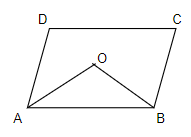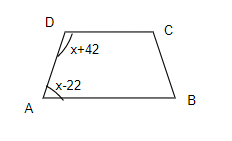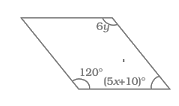# Understanding Quadrilaterals Class 8 Extra Questions

In this page we have Understanding Quadrilaterals Class 8 Extra Questions . Hope you like them and do not forget to like , social share and comment at the end of the page.
Question 1.
The sum of the interior angles of a regular polygon is twice the sum of the exterior angles. Find the number of sides of the polygon

Let n be the sides,then $(n-2) \times 180 = 2 \times 360$ $n=6$

Question 2.
Three angles of the quadrilaterals are in the ratio 2:3:4. The sum of the least and the greatest of these three angles is equal to 180°. Find all the angles of the quadrilateral

Three angles of the quadrilaterals are in the ratio 2:3:4, then it will be 2x,3x,4x.
Now it is given
$2x + 4x=180$
$x=30$
So three angles are 60°,90°,120°
Let y be the fourth angle,Now in a quadrilateral
Sum of all angles =360
$y + 60+90+120=360$
$y=90$
So All the four angles are 60°,90°,120°,90°

Question 3.
The exterior angle of a regular polygon is one-fifth of its interior angle. How many sides has the polygon?

let the interior angle be x,then exterior angle will be (180- x )
Now as per the question
$(180 - x ) = \frac {1}{5} x$
Solving this, we get
x= 150°
Therefore,Each interior angle is 150°
Now we know that
Sum of all interior angles is given by =$( n - 2 ) \times 180$
Therefore
$( n - 2 ) \times 180 = 150 n$
Simplifying this we get
6n - 5n = 12
n = 12
So, Number of sides= 12

Question 4.
One of the angle in the parallelogram is 80°. Find the other angles in the parallelogram

$x + 80=180$
x=100° So angles are 80°,100°,80°, 100°

Question 5.
The angles of the quadrilateral is in the ratio 1:2:3:4. Find all the angles

Let x be common ratio, then angles are 1x,2x,3x,4x
Sum of all angles =360
$1x+2x+3x+4x=360$
$x=36$
So angles are 36°, 72°, 108°,144°

Question 6.
One of the diagonals of a rhombus is equal to its sides. Find the measures of all the angles of the rhombusAlso AB=AC
Now in Triangle ABC,
AB=BC=AC
Hence equliateral triangle
$\angle B=60$, $\angle BAC=60$ and $\angle BCA=60$
Now in Triangle ACD
Hence equliateral triangle
$\angle D=60$, $\angle DAC=60$ and $\angle DCA=60$
Now $\angle A= \angle BAC + \angle DAC=120$
$\angle C =\angle BCA + \angle DCA=120$
Hence angles are 60°, 120°, 60°,120°

Question 7.
In a parallelogram ABCD, the bisectors of ∠ A and ∠ B meet at O. Find ∠ AOB.Question 8.
Find the value of x in the trapezium ABCDSince DC and AB are parallel , these angles are supplementary
$x-22 + x + 42=180$
$2x + 20 =180$
$x=80$

Question 9.
The diagonals of a rhombus are 8 cm and 15 cm. Find its side.

Since diagonals bisect each other and are Perpendicular,Side is given by pythagorus theorem as
$a= \frac {1}{2} \sqrt {d_1^2 + d_2^2} = 8.5$ cm

Question 10.
Find the values of x and y in the following parallelogram.Oppossite angles are equal
So 6y=120, y=20
$120 + 5x + 10=180$
$x=10$

## Summary

This Understanding Quadrilaterals Class 8 Extra Questions is prepared keeping in mind the latest syllabus of CBSE . This has been designed in a way to improve the academic performance of the students. If you find mistakes , please do provide the feebback on the mail. You can download this also using the below link